# segment

Multiple line segment model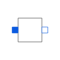# Information

This information is part of the Modelica Standard Library maintained by the Modelica Association.

The segment model is part of the multiple line model. It describes one line segment as outlined in the M_OLine description. Using the loop possibilities of Modelica it is formulated by connecting components the number of which depends on the number of lines.

# Parameters (11)

lines Value: 3 Type: Integer Description: Number of lines Value: div(lines * (lines + 1), 2) Type: Integer Description: Length of the vectors for l, g, c Value: fill(1, dim_vector_lgc) Type: Real[dim_vector_lgc] Description: Capacitance matrix Value: fill(7, lines) Type: Real[lines] Description: Resistance matrix Value: fill(2, dim_vector_lgc) Type: Real[dim_vector_lgc] Description: Inductance matrix Value: fill(1, dim_vector_lgc) Type: Real[dim_vector_lgc] Description: Conductance matrix Value: Type: LinearTemperatureCoefficient (¹/K) Description: Temperature coefficient of resistance (R_actual = R*(1 + alpha*(T_heatPort - T_ref)) Value: Type: LinearTemperatureCoefficient (¹/K) Description: Temperature coefficient of conductance (G_actual = G/(1 + alpha*(T_heatPort - T_ref)) Value: false Type: Boolean Description: =true, if HeatPort is enabled Value: 293.15 Type: Temperature (K) Description: Fixed device temperature if useHeatPort = false Value: Type: Temperature (K)

# Connectors (3)

p n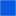Type: PositivePin[lines] Description: Positive pin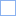Type: NegativePin[lines] Description: Negative pin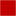Type: HeatPort_a

# Components (5)

C R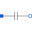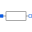Type: Resistor[lines]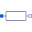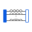Type: M_Transformer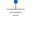Type: Ground

# Used in Components (1)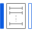M_OLine Modelica.Electrical.Analog.Lines Multiple OLine